# AC Circuit Equations

## Chapter 1 - Useful Equations And Conversion Factors

### Inductive Reactance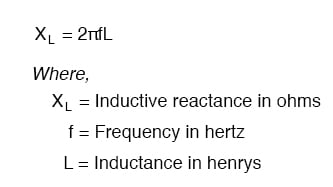### Capacitive Reactance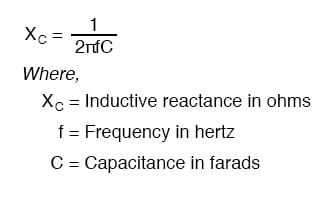ZL = R + jXL

ZC = R - jXC

### Ohm’s Law for AC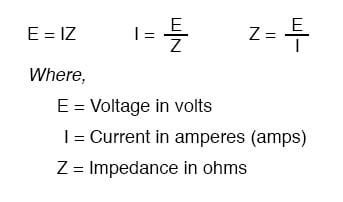### Series and Parallel Impedances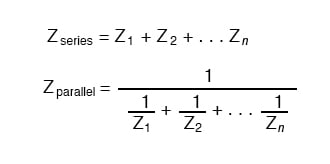NOTE: All impedances must be calculated in complex number form for these equations to work.

### Resonance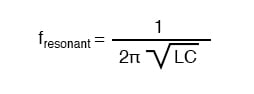NOTE: This equation applies to a non-resistive LC circuit. In circuits containing resistance as well as inductance and capacitance, this equation applies only to series configurations and to parallel configurations where R is very small.

### AC Power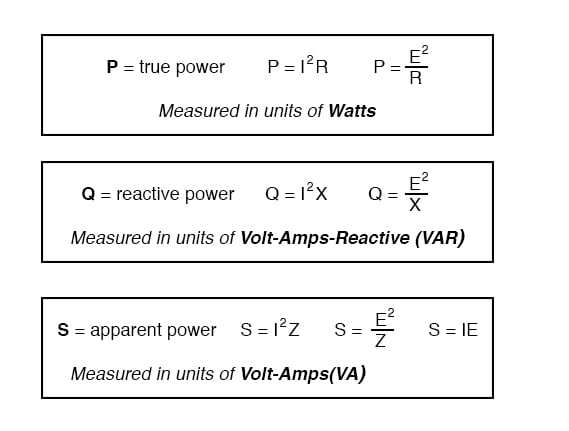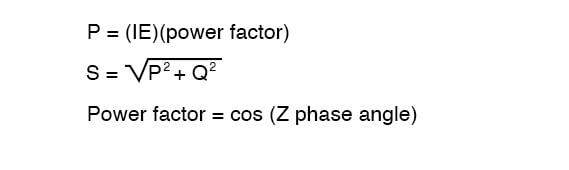RELATED WORKSHEETS:

• Share
Published under the terms and conditions of the Design Science License